# Regularity is hereditary

This article gives the statement, and possibly proof, of a topological space property (i.e., regular space) satisfying a topological space metaproperty (i.e., subspace-hereditary property of topological spaces)
View all topological space metaproperty satisfactions | View all topological space metaproperty dissatisfactions
Get more facts about regular space |Get facts that use property satisfaction of regular space | Get facts that use property satisfaction of regular space|Get more facts about subspace-hereditary property of topological spaces

## Statement

Any subset of a regular space is regular under the subspace topology.

## Definitions used

Fill this in later

## Related facts

### Similar facts

Property Proof that is is subspace-hereditary
Hausdorff space Hausdorffness is hereditary
completely regular space complete regularity is hereditary
Urysohn space Urysohn is hereditary
T1 space T1 is hereditary

Other similar facts:

## Facts used

1. T1 is hereditary

## Proof

Given: A topological space$X$, a subset$A$ of$X$.$X$ is regular.

To prove:$A$ is regular.

Proof: The T1 property for$A$ follows from Fact (1). It thus suffices to show the separation property for$A$, i.e., that any point and disjoint closed subset in$A$ can be separated by a continuous function. In other words, we want to prove the following.

To prove (specific): For any point$x \in A$ and any closed subset$C$ of$A$ such that$x \notin A$, there exist disjoint open subsets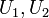$U_1, U_2$ of$A$ such that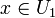$x \in U_1$ and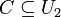$C \subseteq U_2$.

Proof (specific):

Step no. Assertion/construction Facts used Given data used Previous steps used Explanation
1 There exists a closed subset$D$ of$X$ such that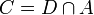$C= D \cap A$. definition of subspace topology$C$ is closed in$A$.
2$x$ is not in$D$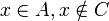$x \in A, x \notin C$ Step (1) [SHOW MORE]
3 There exist disjoint open subsets$V_1, V_2$ of$X$ such that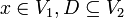$x \in V_1, D \subseteq V_2$.$X$ is regular Steps (1), (2) Step-given combination direct
4 Define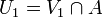$U_1 = V_1 \cap A$ and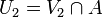$U_2 = V_2 \cap A$.
5$U_1, U_2$ are open subsets of$A$. definition of subspace topology Steps (3), (4) By Step (2),$V_1,V_2$ are open, so by the definition of subspace topology,$U_1, U_2$ are open as per their definitions in Step (3).
6$U_1, U_2$ are disjoint. Steps (3), (4) follows directly from$V_1,V_2$ being disjoint
7$x \in U_1, C \subseteq U_2$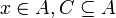$x \in A, C \subseteq A$ Steps (3), (4) By Step (4),$U_1 = V_1 \cap A$. By Step (3),$x \in V_1$, and we are also given that$x\in A$, so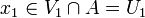$x_1 \in V_1 \cap A = U_1$. Similarly,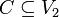$C \subseteq V_2$ and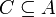$C \subseteq A$, so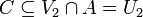$C \subseteq V_2 \cap A = U_2$.
8$U_1, U_2$ are the desired open subsets. Steps (5)-(7) Step-combination, this is exactly what we want to prove.
This proof uses a tabular format for presentation. Learn more about tabular proof formats|View all pages on facts with proofs in tabular format